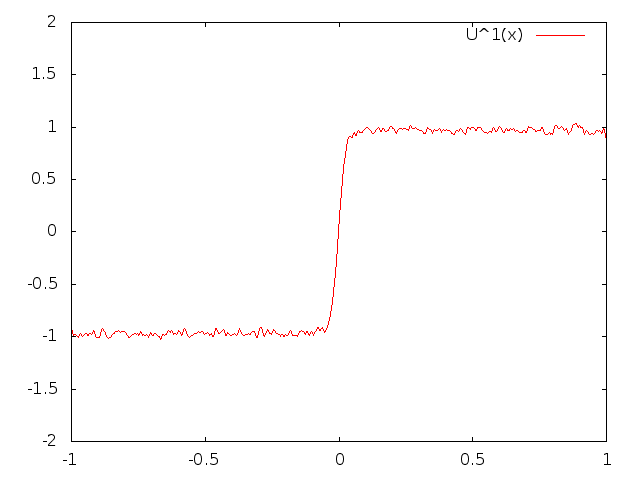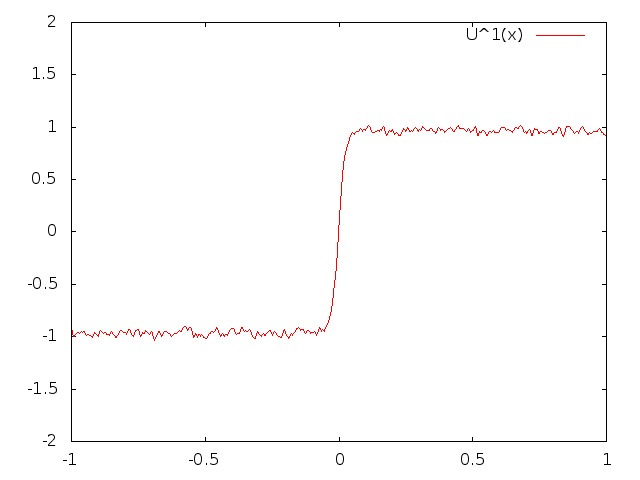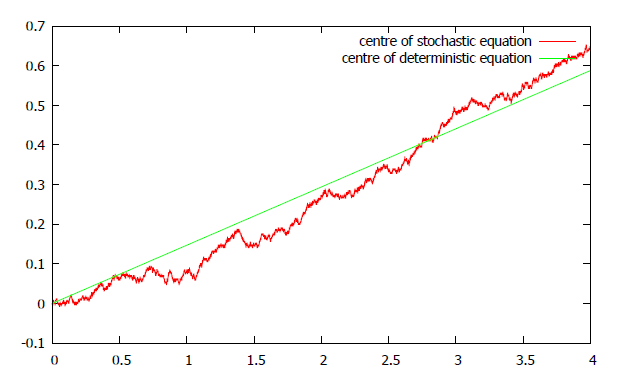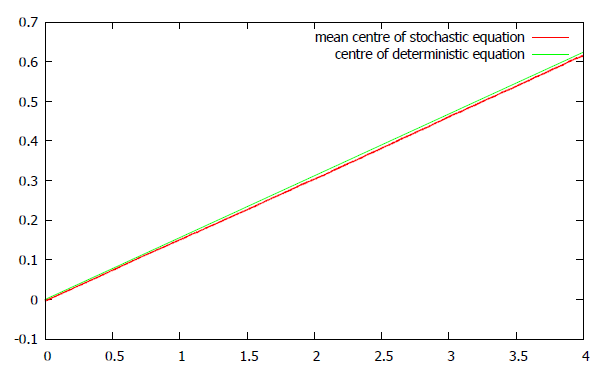# Background: Stochastic Allen-Cahn Equation

We review some basic results on the stochastic Allen-Cahn equation (SACE), which study in $[-\varepsilon^{-1},\varepsilon^{-1}]$ with Neumann boundary conditions and write as

$\displaystyle\frac{\partial u}{\partial t} = \frac{1}{2}\frac{\partial^2 x }{\partial x^2} + f_{\varepsilon}(u) + \sigma \varepsilon^{1/2} \xi$.

Here $f_{\varepsilon}$ is the derivative of a double-well potential, which traditionally was taken to be symmetric.

The first thing to notice is that the equation behaviour changes with the the dimension of the spatial domain. The 1D SACE has a unique continuous weak solution.
The 2D problem is, however, ill-posed, and various ways of making sense of any solutions have been studied.

Probably the best way to get some intuition on the SACE is by showing some numerical experiments of the one-dimensional case. We first consider the Neumann equation with symmetric double well potential, so that $f_{\varepsilon}(u)= u-u^3$.It was proved in Interface Fluctuations and Couplings in the D=1 Ginzburg–Landau Equation with Noise, by S. Brassesco, A. De Masi and E. Presutti, that the point of criticality (that is, the point where the profile in the above animation is zero, see more here), moves according to a Brownian motion in the sharp-interface limit. We conjectured that in the case of an asymmetric double-well potential, resulting on $f_{\varepsilon} = u - u^3 - c\varepsilon (1-u^2)$, the point of criticality should behave like a Brownian motion with a drift paramter $c$. This conjecture was supported by some numerical experiments:
First, in the following animation you can see that, for a given sample path, the point of criticality tends to move to the right.The evolution of the position of the interface over time is shown below, along with that of the corresponding deterministic equation:Below is the sample mean of the centres of 1000 simulated solution paths of the stochastic equation, plotted against the position of the centre of the corresponding deterministic equation.